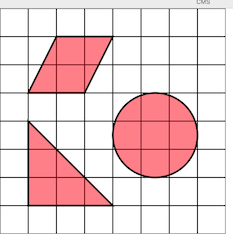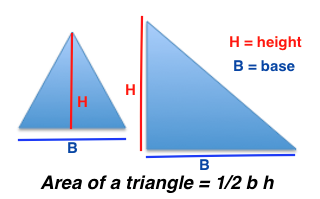# How to Find the Area of a Triangle: Lesson for Kids

Instructor: Trisha Fyfe

Trisha has taught college and K-12 English, reading, writing, and math. She has a master's degree in teaching.

Look around you; measurements are everywhere! We measure the length and height of things all the time. The area of a shape is another important measurement to understand. In this lesson, we will explore the steps to finding the area of triangles.

## What's on the Inside?

Sometimes we need to determine the measurement of the space inside the borders of a shape, not just the distance around the shape. The distance around the edges of a shape is the perimeter. The measurement, or size, of a surface on a shape is called the area. The area includes any space that is inside the borders of this surface. You can find the area of any shape: squares, rectangles, circles, triangles, and even some really funny looking shapes.To find the area of a shape, we need to know which formula to use. Formulas are used all the time in math, and they are really important to know. The formula for finding the area of a triangle is A = ½ bh. This means that we multiply ½ times the base of the triangle (b) times the height of the triangle (h) to get the area of the triangle (A).## A Triangular Sail: What's the Area?

Let's give it a try. Practice makes perfect, right? Say you are measuring out how much fabric will be needed to make a triangular sail for a sailboat. The base for the triangle is 6 feet and the height is 3 feet. Can you figure out how much square feet of fabric will be needed?

While the formula starts with ½ , this doesn't mean we have to start with this number. In multiplication we can multiply the numbers in any order and still get the same answer. So, first let's multiply 6 x 3 = 18. Next, we multiply this number (18) by ½.

Here's the thing though, multiplying any number by ½ just means you are dividing this number by 2. This is helpful to know if you haven't practiced multiplying by fractions quite yet! We divide our first number (18) by 2 (18 ÷ 2 = 9). We need 9 square feet of fabric for this sail.

To unlock this lesson you must be a Study.com Member.

### Register to view this lesson

Are you a student or a teacher?

#### See for yourself why 30 million people use Study.com

##### Become a Study.com member and start learning now.
Back
What teachers are saying about Study.com

### Earning College Credit

Did you know… We have over 200 college courses that prepare you to earn credit by exam that is accepted by over 1,500 colleges and universities. You can test out of the first two years of college and save thousands off your degree. Anyone can earn credit-by-exam regardless of age or education level.